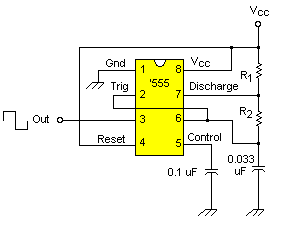# Oscillator

How do you provide a time base for the coilgun logic? What will govern the time duration that each coil is turned on? How do you make an oscillator?

## Oscillator Design

The NE555 (and similar chips) make an excellent oscillator. It is an inexpensive and easy-to-use chip. It can easily be configured for time periods of several seconds to fractions of a millisecond. We'll use an NE555 timer chip to provide a frequency of 13.79 kHz.This is a common arrangement for a free-running oscillator. Capacitor C charges to two-thirds Vcc through R1 and R2, and discharges to one-third Vcc through R2.

The output frequency f is

f = 1.46/(R1 + 2R2)C

where R1,R2 = resistance in ohms
and C = capacitance in farads

Note for reliable operation the resistors should be 1K or larger.

A simplifying assumption is to set R2 = R1 which we'll just call R. This yields the simple formula f = 0.48 / RC. For example, suppose R = 1.0K is a common value in your parts box, and suppose we want f = 13.79 kHz. Then C = 0.033 uF.

Build it and see. It works for me.

By the way, this circuit is not highly frequency stable. The frequency drift is only as good as the capacitor's temperature coefficient. Normal parts may drift several percent with various ambient temperatures. I didn't use a crystal controlled oscillator or NTC parts (negative temperature coefficient) because I won't be firing the coil gun outdoors, and I don't think the frequency is quite that critical anyway. I expect to find one of the biggest factors in reliability will be the exact initial position of the projectile.

 < Previous Page 4 of 16 Next >